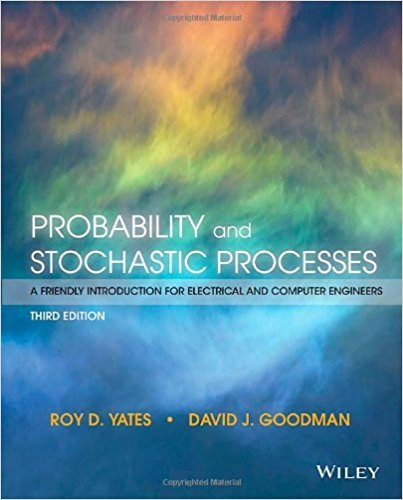×
×

# Solutions for Chapter 8: Random Vectors## Full solutions for Probability and Stochastic Processes: A Friendly Introduction for Electrical and Computer Engineers | 3rd Edition

ISBN: 9781118324561Solutions for Chapter 8: Random Vectors

Solutions for Chapter 8
4 5 0 326 Reviews
20
1
##### ISBN: 9781118324561

This expansive textbook survival guide covers the following chapters and their solutions. This textbook survival guide was created for the textbook: Probability and Stochastic Processes: A Friendly Introduction for Electrical and Computer Engineers, edition: 3. Chapter 8: Random Vectors includes 55 full step-by-step solutions. Since 55 problems in chapter 8: Random Vectors have been answered, more than 11091 students have viewed full step-by-step solutions from this chapter. Probability and Stochastic Processes: A Friendly Introduction for Electrical and Computer Engineers was written by and is associated to the ISBN: 9781118324561.

Key Statistics Terms and definitions covered in this textbook
• 2 k p - factorial experiment

A fractional factorial experiment with k factors tested in a 2 ? p fraction with all factors tested at only two levels (settings) each

• Acceptance region

In hypothesis testing, a region in the sample space of the test statistic such that if the test statistic falls within it, the null hypothesis cannot be rejected. This terminology is used because rejection of H0 is always a strong conclusion and acceptance of H0 is generally a weak conclusion

A formula used to determine the probability of the union of two (or more) events from the probabilities of the events and their intersection(s).

• Alias

In a fractional factorial experiment when certain factor effects cannot be estimated uniquely, they are said to be aliased.

• Analysis of variance (ANOVA)

A method of decomposing the total variability in a set of observations, as measured by the sum of the squares of these observations from their average, into component sums of squares that are associated with speciic deined sources of variation

• Attribute

A qualitative characteristic of an item or unit, usually arising in quality control. For example, classifying production units as defective or nondefective results in attributes data.

• Box plot (or box and whisker plot)

A graphical display of data in which the box contains the middle 50% of the data (the interquartile range) with the median dividing it, and the whiskers extend to the smallest and largest values (or some deined lower and upper limits).

• Components of variance

The individual components of the total variance that are attributable to speciic sources. This usually refers to the individual variance components arising from a random or mixed model analysis of variance.

• Convolution

A method to derive the probability density function of the sum of two independent random variables from an integral (or sum) of probability density (or mass) functions.

• Covariance

A measure of association between two random variables obtained as the expected value of the product of the two random variables around their means; that is, Cov(X Y, ) [( )( )] =? ? E X Y ? ? X Y .

• Deming

W. Edwards Deming (1900–1993) was a leader in the use of statistical quality control.

• Dispersion

The amount of variability exhibited by data

• Error sum of squares

In analysis of variance, this is the portion of total variability that is due to the random component in the data. It is usually based on replication of observations at certain treatment combinations in the experiment. It is sometimes called the residual sum of squares, although this is really a better term to use only when the sum of squares is based on the remnants of a model-itting process and not on replication.

• Error variance

The variance of an error term or component in a model.

• Estimate (or point estimate)

The numerical value of a point estimator.

• Experiment

A series of tests in which changes are made to the system under study

• Exponential random variable

A series of tests in which changes are made to the system under study

• Factorial experiment

A type of experimental design in which every level of one factor is tested in combination with every level of another factor. In general, in a factorial experiment, all possible combinations of factor levels are tested.

• Forward selection

A method of variable selection in regression, where variables are inserted one at a time into the model until no other variables that contribute signiicantly to the model can be found.

• Gaussian distribution

Another name for the normal distribution, based on the strong connection of Karl F. Gauss to the normal distribution; often used in physics and electrical engineering applications

×